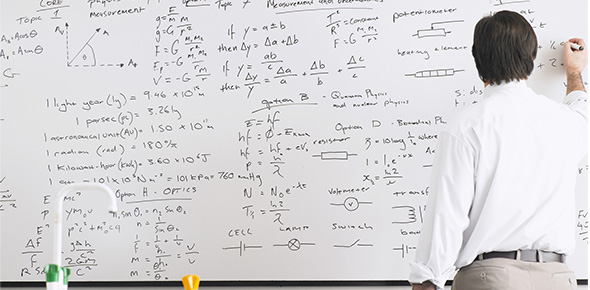# Solving One Step Equations Containing Integers Quiz!

10 QuestionsSettingsAn integer is a number that has no fractional part and no digits after the decimal point. This quiz has been created for students and learners to learn the basic concepts of solving one-step equations including Multiplication, Division, Addition, and Subtraction. Read the questions carefully and answer. Read the questions carefully and answer. So, let's try out the quiz. All the best!

Related Topics
• 1.
H - 15 = -17
• A.

H = -2

• B.

H = 2

• C.

H = 39

• D.

H = -39

• 2.
A - 29 = -12
• A.

A = 41

• B.

A = -41

• C.

A = 17

• D.

A = -17

• 3.
13 = g - 20
• A.

G = 7

• B.

G = 33

• C.

G = -7

• D.

G = -33

• 4.
M + 48 = 31
• A.

M = 79

• B.

M = 17

• C.

M = -79

• D.

M = -17

• 5.
0 = z + 50
• A.

Z = 50

• B.

Z = 0

• C.

Z = -50

• D.

Z = -100

• 6.
15y = -75
• A.

Y = 5

• B.

Y = -60

• C.

Y = -5

• D.

Y = 60

• 7.
0 = -7d
• A.

D = 0

• B.

D = 1

• C.

D = -1

• D.

D = 7

• 8.
R / 4 = -250
• A.

R = 100

• B.

R= -100

• C.

R = 1000

• D.

R = -1000

• 9.
T / -11 = 11
• A.

T = -1

• B.

T = -121

• C.

T = 11

• D.

T = -11

• 10.
Compare the following Integers. -6 _______-20
• A.

<

• B.

>

• C.

=

• D.

None of the above